• BRDF
2019-10-21 20:43:56

BRDF分为漫反射和高光反射部分

漫反射部分

unity采用的是Disney漫反射模型

half DisneyDiffuse(half NdotV, half NdotL, half LdotH, half roughness)

{

half nlPow5 = Pow5 (1-NdotL);

half nvPow5 = Pow5 (1-NdotV);

half Fd90 = 0.5 + 2 * LdotH * LdotH * roughness;

half disneyDiffuse = (1 + (Fd90-1) * nlPow5) * (1 + (Fd90-1) * nvPow5);

half diffuseTerm = disneyDiffuse * NdotL;

return diffuseTerm;

}

高光反射部分

spec term比较多，分GDF三大部分

//G

//------------------------------------------------------------------------------------------------------------------------

float ImplicitGeometricShadowingFunction (float NdotL, float NdotV){

float Gs = (NdotL*NdotV);

return Gs;

}

float AshikhminShirleyGeometricShadowingFunction (float NdotL, float NdotV, float LdotH){

float Gs = NdotL*NdotV/(LdotH*max(NdotL,NdotV));

return (Gs);

}

float AshikhminPremozeGeometricShadowingFunction (float NdotL, float NdotV){

float Gs = NdotL*NdotV/(NdotL+NdotV - NdotL*NdotV);

return (Gs);

}

float DuerGeometricShadowingFunction (float3 lightDirection,float3 viewDirection, float3 normalDirection,float NdotL, float NdotV){

float3 LpV = lightDirection + viewDirection;

float Gs = dot(LpV,LpV) * pow(dot(LpV,normalDirection),-4);

return (Gs);

}

float NeumannGeometricShadowingFunction (float NdotL, float NdotV){

float Gs = (NdotL*NdotV)/max(NdotL, NdotV);

return (Gs);

}

float KelemenGeometricShadowingFunction (float NdotL, float NdotV, float LdotH, float VdotH){

// float Gs = (NdotL*NdotV)/ (LdotH * LdotH); //this

float Gs = (NdotL*NdotV)/(VdotH * VdotH); //or this?

return (Gs);

}

float ModifiedKelemenGeometricShadowingFunction (float NdotV, float NdotL, float roughness)

{

float c = 0.797884560802865; // c = sqrt(2 / Pi)

float k = roughness * roughness * c;

float gH = NdotV * k +(1-k);

return (gH * gH * NdotL);

}

float CookTorrenceGeometricShadowingFunction (float NdotL, float NdotV, float VdotH, float NdotH){

float Gs = min(1.0, min(2*NdotH*NdotV / VdotH, 2*NdotH*NdotL / VdotH));

return (Gs);

}

float WardGeometricShadowingFunction (float NdotL, float NdotV, float VdotH, float NdotH){

float Gs = pow( NdotL * NdotV, 0.5);

return (Gs);

}

float KurtGeometricShadowingFunction (float NdotL, float NdotV, float VdotH, float alpha){

float Gs = (VdotH*pow(NdotL*NdotV, alpha))/ NdotL * NdotV;

return (Gs);

}

//F

//------------------------------------------------------------------------------------------------------------------------

//schlick functions

float SchlickFresnel(float i){

float x = clamp(1.0-i, 0.0, 1.0);

float x2 = x*x;

return x2*x2*x;

}

float3 FresnelLerp (float3 x, float3 y, float d)

{

float t = SchlickFresnel(d);

return lerp (x, y, t);

}

float3 SchlickFresnelFunction(float3 SpecularColor,float LdotH){

return SpecularColor + (1 - SpecularColor)* SchlickFresnel(LdotH);

}

float SchlickIORFresnelFunction(float ior,float LdotH){

float f0 = pow((ior-1)/(ior+1),2);

return f0 + (1 - f0) * SchlickFresnel(LdotH);

}

float SphericalGaussianFresnelFunction(float LdotH,float SpecularColor)

{

float power = ((-5.55473 * LdotH) - 6.98316) * LdotH;

return SpecularColor + (1 - SpecularColor) * pow(2,power);

}

//D

//------------------------------------------------------------------------------------------------------------------------

//Normal Distribution Functions

float BlinnPhongNormalDistribution(float NdotH, float specularpower, float speculargloss){

float Distribution = pow(NdotH,speculargloss) * specularpower;

Distribution *= (2+specularpower) / (2*3.1415926535);

return Distribution;

}

float PhongNormalDistribution(float RdotV, float specularpower, float speculargloss){

float Distribution = pow(RdotV,speculargloss) * specularpower;

Distribution *= (2+specularpower) / (2*3.1415926535);

return Distribution;

}

float BeckmannNormalDistribution(float roughness, float NdotH)

{

float roughnessSqr = roughness*roughness;

float NdotHSqr = NdotH*NdotH;

return max(0.000001,(1.0 / (3.1415926535*roughnessSqr*NdotHSqr*NdotHSqr))* exp((NdotHSqr-1)/(roughnessSqr*NdotHSqr)));

}

float GaussianNormalDistribution(float roughness, float NdotH)

{

float roughnessSqr = roughness*roughness;

float thetaH = acos(NdotH);

return exp(-thetaH*thetaH/roughnessSqr);

}

float GGXNormalDistribution(float roughness, float NdotH)

{

float roughnessSqr = roughness*roughness;

float NdotHSqr = NdotH*NdotH;

float TanNdotHSqr = (1-NdotHSqr)/NdotHSqr;

return (1.0/3.1415926535) * sqr(roughness/(NdotHSqr * (roughnessSqr + TanNdotHSqr)));

// float denom = NdotHSqr * (roughnessSqr-1)

}

float TrowbridgeReitzNormalDistribution(float NdotH, float roughness){

float roughnessSqr = roughness*roughness;

float Distribution = NdotH*NdotH * (roughnessSqr-1.0) + 1.0;

return roughnessSqr / (3.1415926535 * Distribution*Distribution);

}

float TrowbridgeReitzAnisotropicNormalDistribution(float anisotropic, float NdotH, float HdotX, float HdotY){

float aspect = sqrt(1.0h-anisotropic * 0.9h);

float X = max(.001, sqr(1.0-_Glossiness)/aspect) * 5;

float Y = max(.001, sqr(1.0-_Glossiness)*aspect) * 5;

return 1.0 / (3.1415926535 * X*Y * sqr(sqr(HdotX/X) + sqr(HdotY/Y) + NdotH*NdotH));

}

float WardAnisotropicNormalDistribution(float anisotropic, float NdotL, float NdotV, float NdotH, float HdotX, float HdotY){

float aspect = sqrt(1.0h-anisotropic * 0.9h);

float X = max(.001, sqr(1.0-_Glossiness)/aspect) * 5;

float Y = max(.001, sqr(1.0-_Glossiness)*aspect) * 5;

float exponent = -(sqr(HdotX/X) + sqr(HdotY/Y)) / sqr(NdotH);

float Distribution = 1.0 / ( 3.14159265 * X * Y * sqrt(NdotL * NdotV));

Distribution *= exp(exponent);

return Distribution;

}

更多相关内容
• 软件介绍: brdf explorer是一迪士尼开发的brdf的浏览工具，功能非常强大，这个是V1.0版本，绿色软件不用安装。能够以各种视图模式下查看。
•matlab
• pbrt-重要性-采样 PBRT 扩展对 brdf 数据使用重要性采样算法用于数据压缩（brdf 功能）：Douglas PeuckerC++
• 研究了沙漠地区地表起伏程度及太阳天顶角对BRDF 的影响，结果表明，在太阳几何一定的情况下，不同起伏地形的BRDF 随观测方位角的变化呈不对称分布，且近垂直主平面方向的BRDF 比近主平面方向整体偏大；对于同一地形...
• BRDF模型介绍得非常详细，photemetric stereo多光技术的非朗伯反射方法大多基于BRDF模型，有了一个清晰的认识
• matlab集成c代码 /系统各模块说明*****/ 一、系统初始化 1、材质台就位 2、相机、光源、材质台控制测试 二、预处理 1、工业相机标定及校正预处理 ...8）逐角度计算图像平均亮度/逐像素亮度，保存BRDF材质数据
• 提出了一种快速测量双向反射分布函数(BRDF)的子孔径扫描傅里叶变换系统。子孔径扫描傅里叶变换方法,使用单个傅里叶变换透镜进行测量,具有扩展单次测量视场、工作距离长、成本低以及系统简单等优点,可以在大空间频率...
• 在此将褶皱看作一种材质,提出基于宏观光学散射截面测量的双向反射分布函数(BRDF)生成方法,求得褶皱材质的BRDF数据,进一步利用误差逆传播(BP)神经网络建立了褶皱材质的BRDF模型,代替了复杂的褶皱建模过程,大大简化了...
• 由于双向反射分布函数( BRDF)经验模型与半经验模型对材质散射特性描述时存在局限性，导致其拟合结果与实测数据的误差较大。针对此问题，基于深度神经网络(DNN)构建了一种适用于具有不同散射特性空间目标材质的BRDF...
• 将均匀度指数引入林木榨间分布格对双向反射分布函数（BRDF）影响的分析中，利川光学遥感模型定最分析林木空间分布格局对BRDF的影响、结果表明：对于红光波段，在大于树冠尺度上均匀度口以以有效地描述林木空间分布局...
• 开发这些程序是为了利用 Eldim 开发的 EZ-Contrast 仪器的亮度或 BRDF 数据。 它们适用于使用 ccd 传感器进行的任何测量。 开发的库包括： - 最大值研究- ccd 截面的极坐标天顶图- ccd 截面的极坐标图- 根据天顶角...matlab
• 对目标表面双向反射分布函数(BRDF)进行研究，在各向异性Gaussian模型的基础上引入波长因子，提出了改进的BRDF各向异性Gaussian计算模型。运用该模型对卫星太阳能板及包裹多层隔热材料进行了BRDF计算，结果表明该模型...
• 非常好的brdf的浏览工具，迪士尼开发，超强大，非常好用
• 文章目录光照、照明（Illumination）预备知识球面坐标（Spherical Coordinate）立体角（Solid ...BRDF的定义与性质BRDF 的定义可逆性（Reciprocity）能量守恒性质渲染方程（Rendering Equation）BRDF模型经验模型（Em

双向反射分布函数（BRDF：Bidirectional Reflectance Distribution Function），描述的是物体表面对光的反射性质。

### 光照、照明（Illumination）

光照可分为局部和全局两种
局部光照（local illumination） 和物体直接被光源照射的情况相关
全局光照（global illumination） 和物体被光源以外的其它地方来的光线照射的情况相关，包括其它物体反射和投射过来的光线。

今天的主题：一个关于光线如何被表面反射的物理描述，也就是 BRDF。

### 预备知识

球面坐标（Spherical Coordinate）
立体角（Solid Angle）
投影面积（Foreshortened Area）
发光强度（Intensity）

#### 球面坐标（Spherical Coordinate）

由于光线主要是通过方向来表达，通常用球面坐标表达它们比用笛卡尔坐标更方便。
如图所示，球面坐标中的向量用三个元素来指定：
r r 表示向量的长度；
θ \theta 表示向量和 z z 轴的夹角；
ϕ \phi 表示向量在 x − y x-y 平面上的投影和 x x 轴的逆时针夹角。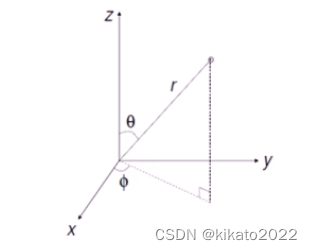球面坐标与三维笛卡尔坐标（Cartesian Coordinates）之间的对应关系：

C a r ( x , y , z ) = S p h ( r , θ , ϕ ) Car(x, y, z) = Sph(r, \theta, \phi)

它们的转换公式为：
r = x 2 + y 2 + z 2 θ = arccos ⁡ ( z / r ) ϕ = arctan ⁡ ( y / x ) \begin{aligned} r &= \sqrt{x^2 + y^2 + z^2} \\ \theta &= \arccos(z/r) \\ \phi &= \arctan(y/x) \end{aligned}

z = r cos ⁡ ( θ ) y = r sin ⁡ ( θ ) s i n ( ϕ ) x = r sin ⁡ ( θ ) c o s ( ϕ ) \begin{aligned} z &= r \cos (\theta) \\ y &= r \sin (\theta) sin(\phi) \\ x &= r \sin (\theta) cos(\phi) \end{aligned}

#### 立体角（Solid Angle）

立体角描述了从原点向一个球面区域张成的视野大小，是平面角在三维的自然推广。立体角的最大值为全角： 4 π 4\pi ，该最大值可以在区域为整个球面时取到。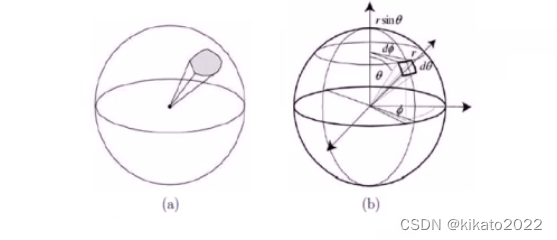立体角 ω \omega 具有如下微分形式：
d ω = d A r 2 d \omega = \frac{d A}{r^2}
由于面积微元在球面坐标系下可以写成：
d A = ( r d θ ) ( r sin ⁡ θ d ϕ ) = r 2 sin ⁡ θ d θ d ϕ dA = (rd\theta)(r \sin \theta d\phi)=r^2\sin\theta d\theta d\phi
因此：
d ω = d A r 2 = sin ⁡ θ d θ d ϕ d\omega = \frac{dA}{r^2}=\sin \theta d\theta d\phi

#### 投影面积（Foreshortened Area）

投影面积描述了一个物体表面的微小区域在某个视线方向上的可见面积。对于面积微元 A A ，则沿着与法相夹角为 θ \theta 方向的 A A 的可见面积为:
a r e a = A cos ⁡ θ area = A \cos \theta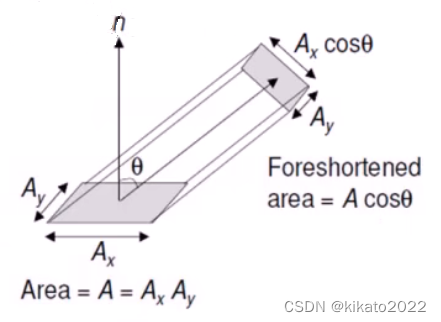光能表示的是（一个区域中）光子能量的总和。光能通常使用符号 Q Q 来表示，其单位是焦耳（J）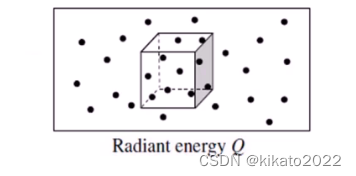光能并不会停留和储存在某个位置，而是在始终不断运动着的。光通量描述的是在单位时间穿过截面的光能（通常用符号 Φ \Phi 来表示，单位是瓦特 W W
Φ = d Q d t \Phi = \frac{dQ}{dt}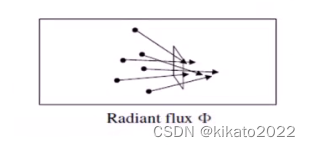辉度表示的是物体表面受光能的影响程度，它等于单位面积上的光通量（通常用符号 E E 来表示，单位是 W / m 2 W/m^2
E = d Φ d A E=\frac{d\Phi}{dA}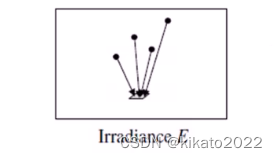#### 发光强度（Intensity）

对一个点（例如点光源）来说，发光强度表示单位立体角上的光通量（发光强度简称光强，使用符号 I I 来表示，单位是 W W
I = d Φ d ω I=\frac{d\Phi}{d\omega}光亮度表示物体表面沿某一方向的明亮程度，它等于单位投影面积在单位立体角上的光通量，使用符号 L L 来表示，单位是 W / m 2 W/m^2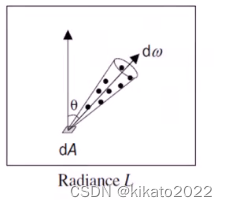一种直观的光亮度的理解方法是：将光亮度理解为物体表面的微面元所接收的来自于某方向光源的单位面积的光通量，因此截面选用垂直于该方向的截面，其面积按投影面积计算。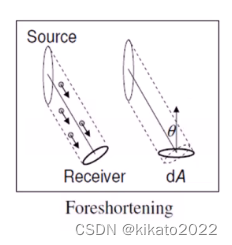光亮度的微分形式：
L = d Φ d A cos ⁡ θ d ω L=\frac{d\Phi}{dA\cos\theta d \omega}
光亮度使用物体表面沿目标方向上的投影面积，而不是面积

#### 辉度和光亮度的关系

辉度可以写成光亮度在入射光所形成的半球上的积分：
d Φ d A = E = ∫ Ω L ( ω ) cos ⁡ θ ( ω ) d ω \frac{d\Phi}{dA} = E = \int_{\Omega}L(\omega) \cos \theta(\omega) d\omega

• Ω \Omega 是入射光所形成的半球
• L ( ω ) L(\omega) 是沿 ω \omega 方向的光亮度

### BRDF的定义与性质

BRDF 的定义
BRDF 的性质：
- 可逆性（Reciprocity）
- 能量守恒性质
- 基于 BRDF 的渲染方程（Rendering Equation）

#### BRDF 的定义

BRDF 描述的是物体表面将光能从任何一个入射方向反射到任何一个视点方向的反射特性。BRDF 模型是绝大多数近代图形学算法中用于描述光反射现象的基本模型。
BRDF 是关于入射光方向和反射光方向的四维实值函数，它等于反射方向的光亮度和沿入射方向的入射光的辉度之比：
f ( ω i → ω r ) = d L r ( ω r ) d E i f(\omega_i \rightarrow \omega_r) = \frac{dL_r(\omega_r)}{dE_i}
可以写成关于入射光的光亮度的形式：
f ( ω i → ω r ) = d L r ( w r ) L i ( ω i ) cos ⁡ ω i d ω i f(\omega_i \rightarrow \omega_r)=\frac{dL_r(w_r)}{L_i(\omega_i)\cos \omega_i d\omega_i}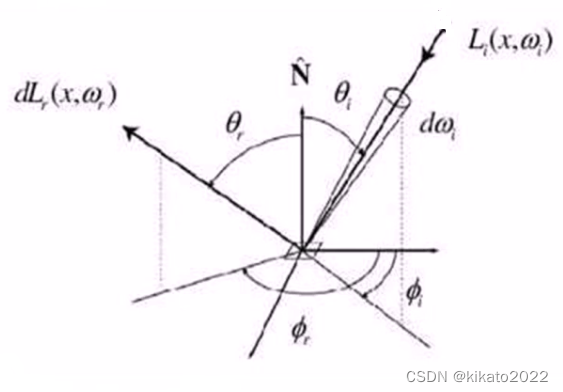#### 可逆性（Reciprocity）

BRDF 的可逆性源自于 Helmholtz 光路可逆性（Helmholtz Reciprocity Rule）
BRDF 的可逆性是说：交换入射光与反射光的角色，并不会改变 BRDF 的值：
f ( ω i → ω r ) = f ( ω r → ω i ) f(\omega_i \rightarrow \omega_r)=f(\omega_r \rightarrow \omega_i)

#### 能量守恒性质

BRDF 需要遵循的另一个物理定律是能量守恒定律。能量守恒定律指出：入射光的能量与出射光的总能量应该相等。
能量守恒方程如下：
Q i n c o m i n g = Q r e f l e c t e d + Q a b s o r b + Q t r a n s m i t t e d Q_{incoming}=Q_{reflected}+Q_{absorb}+Q_{transmitted}
由此我们知道：
Q r e f l e c t e d ≤ Q i n c o m i n g Q_{reflected} \leq Q_{incoming}
因此 BRDF 必须满足如下的积分不等式，也就是所谓的能量守恒性质：
∫ Ω f ( ω i → ω r ) cos ⁡ ω r d ω r ≤ 1 \int_{\Omega}f(\omega_i \rightarrow \omega_r) \cos \omega_r d \omega_r \leq 1

#### 渲染方程（Rendering Equation）

渲染方程（Rendering Equation）用于计算环境光照明下的反射光的光亮度，它可以写成不同角度下入射光的光亮度乘以 BRDF 的积分：
L r = ∫ Ω f ( ω i → ω r ) L ( ω i ) cos ⁡ ω i d ω i L_r = \int_{\Omega}f(\omega_i \rightarrow \omega_r)L(\omega_i)\cos \omega_i d\omega_i

#### BRDF模型

为了方便和高效地使用 BRDF 数据，它们往往被组织成为参数化的数值模型。
BRDF 的数值模型具有如下三类：
- 经验模型（Empirical Models）
- 基于物理的模型（Physical-based Models）
- 数据表达的模型（Data-driven Models）

• 经验模型，使用基于实验提出的公式对 BRDF 做快速的估计
• 基于物理的模型，根据物体表面材料的几何以及光学属性建立反射方程，从而计算 BRDF
• 数据表达的模型，将 BRDF 按照实测数据建立查找表，以便于快速的查找和计算。
##### 经验模型（Empiriccal Models）

经验模型提供简洁的公式以便于反射光线的快速计算；
经验模型不考虑材质特性，仅仅提供一个反射光的粗糙近似。
经验模型不一定满足物理定律，如 Helmholtz 可逆性或能量守恒定律等。
经验模型因为其简洁和高效性被广泛运用。

###### 经验模型1：Lambertian 模型
• Lambertian模型是最基本的反射模型：
- 入射光线被均匀地反射到各个方向。
- 沿不同方向地 BRDF 是一个常数。
• 反射率（Albedo）： ρ \rho
- 反射率是反射光辉度与入射光辉度之比。
L r ( ω r ) = ∫ Ω f L i ( ω i ) cos ⁡ ω i d ω i = f ∫ ω L i ( ω i ) cos ⁡ ω i d ω i = f E i \begin{aligned} L_r(\omega_r) &= \int _{\Omega}f L_i(\omega_i)\cos \omega_i d\omega_i\\ &= f\int_{\omega}L_i(\omega_i) \cos \omega_i d\omega_i \\ &= fE_i \end{aligned}
ρ = E r E i = ∫ Ω L r ( ω r ) cos ⁡ ω r d ω r E i = f E i π E i = π f \rho = \frac{E_r}{E_i}=\frac{\int_\Omega L_r(\omega_r)\cos \omega_r d\omega_r}{E_i}=\frac{fE_i \pi}{E_i} = \pi f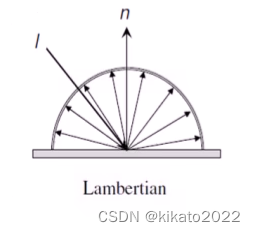该模型能够很好地用于描述那些包含存粹漫反射的物体（例如：纸张）。
Lambertian 漫反射模型不能表现出材质的镜面反射效果，而镜面反射对于金属材质非常重要。
由于 Lambertian 模型的简洁以及对漫反射良好的描述特性，它常常在其它的经验模型（例如：Phong 模型）中作为分量的形式被包含。

###### 经验模型2：Phong模型

Phong 模型是在 Lambertian 漫反射模型的基础上，添加了一项镜面反射项，以表达在反射角上的镜面反射效果：

f ( l → v ) = ρ d + ρ s ( r ⋅ v ) s ( n ⋅ l ) f(l\rightarrow v) = \rho_d + \rho_s \frac{(r\cdot v)^s}{(n\cdot l)}
其中， ρ d \rho_d ρ s \rho_s 分别表示漫反射光和镜面反射光线的反射率， s s 表示发光指数，用于描述镜面反射的锐利度。

Phong 模型不满足可逆性：
f ( l → v ) ≠ f ( v → l ) f(l\rightarrow v) \neq f(v\rightarrow l)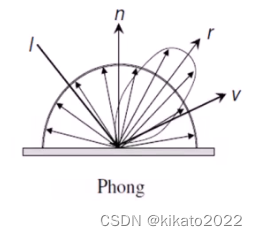尽管 Phong 模型缺乏物理解释，并且对于某些金属材质，它并不十分准确；但 Phong 模型仍是目前计算机图形学中被最为广泛使用的基于经验的反射模型。

Phong 模型的优势在于它的简洁和高效性，以及能够同时表现漫反射和镜面反射的特征。

###### Phong 模型的扩展

Phong 模型的大部分扩展是为了实现进一步的加速。

Blinn-Phong 模型
- 通过引入入射方向 l l 和视点方向 v v 的角平分线 h h ，使用 h h 和法向 n n 的点积替代原先 Phong 模型中 r r v v 的点积，可以简化运算：
f ( l → v ) = ρ d + ρ s ( n ⋅ h ) s ( n ⋅ l )    w h e r e   h = v + l 2 f(l\rightarrow v) = \rho_d + \rho_s \frac{(n\cdot h)^s}{(n\cdot l)}\ \ where\ h=\frac{v+l}{2}

由 Bishop 和 Weimer 发表在 1986年 的 ACM SIGGRAPH 年会上。
思想是使用制表和插值的方法对指数项 ( r ⋅ v ) s (r\cdot v)^s 进行快速计算，从而实现绘制的加速。

可逆的 Phong 模型（Modified Phong Model）
通过去掉 Phong 模型中镜面反射分量的分母项，从而使得修改后的 Phong 模型能够满足可逆性：
f ( l → v ) = ρ d + ρ s ( r ⋅ v ) s f ( l → v ) = f ( v → l ) \begin{aligned} f(l \rightarrow v) &= \rho_d+\rho_s(r\cdot v)^s\\ f(l \rightarrow v) &= f(v \rightarrow l) \end{aligned}
Phong模型的示例效果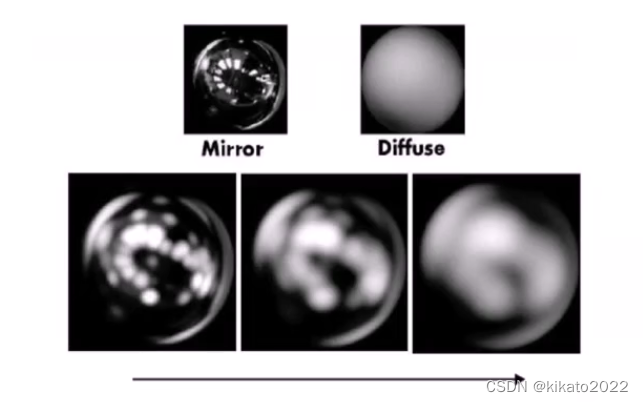##### 物理模型（Physical-Based Models）

- 经验模型（Empirical Models）源于设计者的直觉和实践经验，而物理模型则建立在有关光的相互作用的科学知识上。通过包含材料的各种几何及光学性质来尽可能精确的近似现实世界中的材料。
- 物理模型（Physical-Based Models）通常建立在被成为表面粗糙度（surface roughness）的细节几何结构上。
粗糙度：从微观角度来看，几乎没有完全光滑的表面；微观尺度的表面几何是通过一组微平面（microfacets）集合来建模的；粗糙度通过微平面法向的统计分布来表达的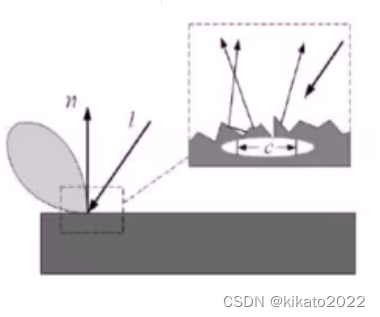菲涅尔项（Fresnel Term）
在实际应用中，我们发现，单向反射性在擦地角（grazing angles）附近增大入射光的反射量是由从麦克斯韦电磁波方程组中得到的菲涅尔公式得到的。

菲涅尔项（Fresnel Term）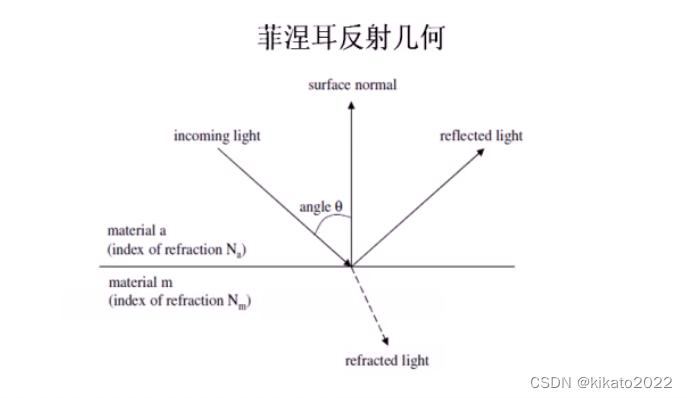定义如下：
F s = a 2 + b 2 − 2 a cos ⁡ θ + cos ⁡ 2 θ a 2 + b 2 + 2 a cos ⁡ θ + cos ⁡ 2 θ F p = F s a 2 + b 2 − 2 a sin ⁡ θ tan ⁡ θ + sin ⁡ 2 θ tan ⁡ 2 θ a 2 + b 2 + 2 a sin ⁡ θ tan ⁡ θ + sin ⁡ 2 θ tan ⁡ 2 θ 2 a 2 = ( η 2 − κ 2 − sin ⁡ 2 θ ) 2 + 4 η 2 κ 2 + ( η 2 − κ 2 − sin ⁡ 2 θ ) 2 b 2 = ( η 2 − κ 2 − sin ⁡ 2 θ ) 2 + 4 η 2 κ 2 − ( η 2 − κ 2 − sin ⁡ 2 θ ) η = η m η a + κ m κ a η a 2 + κ a 2 κ = η a κ m − η m κ a η a 2 + κ a 2 \begin{aligned} F_s &= \frac{a^2+b^2-2a\cos\theta +\cos^2\theta}{a^2+b^2+2a\cos\theta +\cos^2\theta}\\ F_p &= F_s\frac{a^2+b^2-2a\sin\theta\tan\theta+\sin^2\theta\tan^2\theta}{a^2+b^2+2a\sin\theta\tan\theta+\sin^2\theta\tan^2\theta} \\ 2a^2 &= \sqrt{(\eta^2-\kappa^2-\sin^2\theta)^2+4\eta^2\kappa^2} +(\eta^2 - \kappa^2 - \sin^2 \theta) \\ 2b^2 &= \sqrt{(\eta^2-\kappa^2-\sin^2\theta)^2+4\eta^2\kappa^2} -(\eta^2 - \kappa^2 - \sin^2 \theta)\\ \eta &= \frac{\eta_m\eta_a+\kappa_m\kappa_a}{\eta^2_a+\kappa^2_a}\\ \kappa &= \frac{\eta_a\kappa_m-\eta_m \kappa_a}{\eta^2_a+\kappa^2_a} \end{aligned}
菲涅耳反射率： F = F p + F s 2 F=\frac{F_p+F_s}{2}

###### Cook-Torrance 模型

由 Cook 和 Torrance 提出[ A Reflectance Model for Computer Graphics, ACM SIGGRAPH, 1981]
在图形学中使用最早的 BRDF 物理模型
是应用物理学家开发的 Torrance-Sparrow 模型的一个应用版本。[Theory for Off-Specular Reflection from Roughened Surfaces, J.Optical Society of America, 1975]

假设微平面是镜面反射的
微平面被假定按如图所示 V V 形沟槽排列。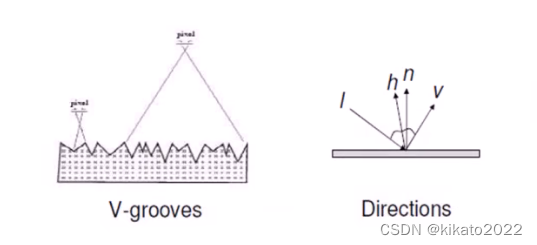结合 Lambertian 漫反射项与微平面反射的镜面反射项：
f λ = d ρ d π + s F λ D G 4 π ( n ⋅ l ) ( n ⋅ v ) f_\lambda = d\frac{\rho_d}{\pi}+s F_\lambda\frac{DG}{4\pi(n\cdot l)(n\cdot v)}
这里的 F λ F_\lambda 是菲涅尔因子， D D 是微平面法向的分布函数， G G 是几何衰减因子， s s , d d 是镜面反射和漫反射系数。

微平面法向的分布函数 D D
由于微平面只有镜面反射，只有那些法向沿着平分线方向 h h 的才对镜面反射起作用。
采用 Bechmann 分布来描述：
D ( h ) = 1 m 2 cos ⁡ 4 β e − tan ⁡ 2 β m 2 D(h) = \frac{1}{m^2\cos^4\beta}e^{-\frac{\tan^2\beta}{m^2}}
其中， m m 是表面的粗糙度值， β \beta n n h h 的夹角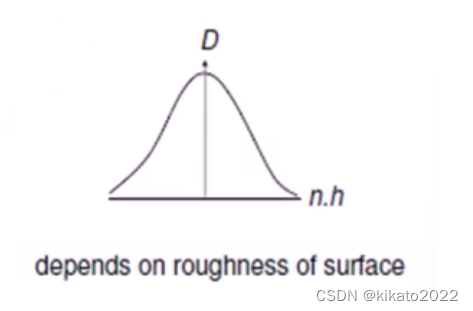几何衰减系数 G G (Geometric Attenuation Factor)
考虑来自视角方向的遮挡效果和来自光线方向的阴影效果
G （ n , l , v ) = min ⁡ { 1 , 2 ( n ⋅ h ) ( n ⋅ v ) ( v ⋅ h ) , 2 ( n ⋅ h ) ( n ⋅ l ) ( v ⋅ h ) } G（n,l,v)=\min \left \{1, \frac{2(n\cdot h)(n\cdot v)}{(v\cdot h)}, \frac{2(n\cdot h)(n\cdot l)}{(v\cdot h)} \right\}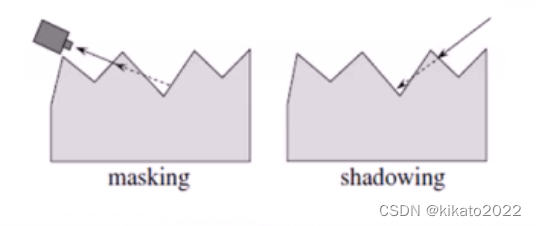这种对微平面遮挡和阴影的考虑使得可以产生某些反射特性
一个是反射方向不在镜面方向的反射（off-specular reflection）
另一个是逆反射（retroreflection）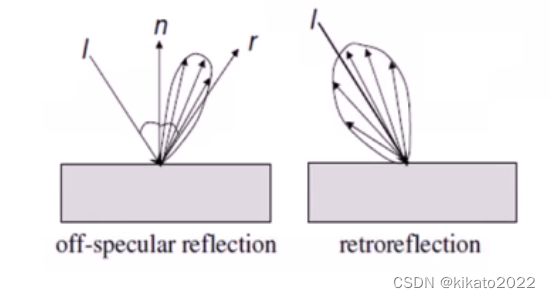反射方向不在镜面方向的反射（Off-specular reflection）
- 反射方向不在镜面反射方向上
- 这是典型的粗糙表面的特征
逆反射（Retroreflection）
- 很大一部分光线沿着反方向返回
- 例如下图中的满月图片，边缘看起来和中心一样明亮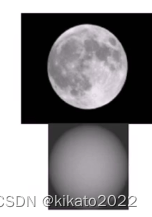Cook-Torrance模型与Phong模型的结果对比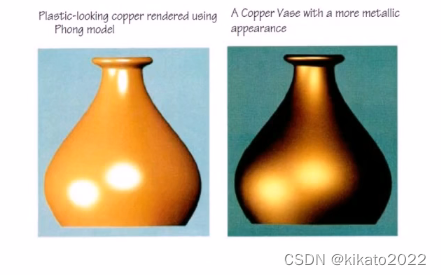更多的 Cook-Torrance 模型示例结果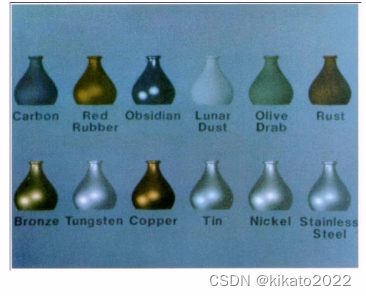###### 其它的物理模型

BRDF可分为两类

• 各向同性（Isotropic）
- 反射不受与给定表面法向夹角的约束
- 随机表面微结构
• 各项异性（Anisotropic）
- 反射比随着与某个给定的表面法向之间的夹角而变化
- 图案的表面微结构
- 金属丝，绸缎，毛发
###### Ward 模型

然而，Phong 和 Cook-Torrance BRDF 模型都不能处理各项异性的效果
现在我们介绍另一种 BRDF 模型：Ward 模型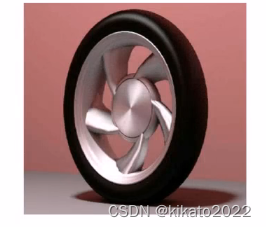- Ward 模型由 Ward 于 1992 年提出[Measuring and Modeling Anisotropic Reflection, ACM SIGGRAPH, 1992]
- 介绍了一种更一般的表面法向表达方式：通过椭圆体（ellispsoids）这种允许各向异性反射的形式来表达
- 然而，由于没有考虑菲涅尔因子（Fresnel factor）和几何衰减因子（Geometric attenuation factor），该模型更像是一种经验模型

各向同性的 Ward 模型定义为：
ρ b d   i s o ( θ i , ϕ i , θ i , ϕ r ) = ρ d π + ρ s ⋅ 1 cos ⁡ θ i cos ⁡ θ r ⋅ e − tan ⁡ 2 σ α 2 4 π α 2 \rho _{bd\ iso}(\theta_i,\phi_i, \theta_i,\phi_r)=\frac{\rho_d}{\pi}+\rho_s \cdot \frac{1}{\sqrt{\cos \theta_i \cos \theta_r}}\cdot \frac{e^{-\tan^2{\frac{\sigma}{\alpha^2}}}}{4\pi \alpha^2}
其中：
ρ d \rho_d 是漫反射系数
ρ s \rho_s 是镜面反射系数
σ \sigma n ^ \hat{n} h ^ \hat{h} 的夹角
α \alpha 是表面坡度的标准差

将菲涅尔因子和几何衰减因子替换为一个用于保证分布在整个半球内积分的简单归一化项。

各向异性的 Ward 模型定义为：
ρ b d ( θ i , ϕ i , θ r , ϕ r ) = ρ d π + ρ s ⋅ 1 cos ⁡ θ i cos ⁡ θ r ⋅ exp ⁡ [ − tan ⁡ 2 σ ( cos ⁡ 2 ϕ / α x 2 + sin ⁡ 2 ϕ / α y 2 ) ] 4 π α x α y \rho_{bd}(\theta_i,\phi_i,\theta_r,\phi_r)=\frac{\rho_d}{\pi}+\rho_s\cdot \frac{1}{\sqrt{\cos \theta_i\cos\theta_r}}\cdot \frac{\exp[-\tan^2\sigma(\cos^2\phi/\alpha_x^2 + \sin^2\phi / \alpha_y^2)]}{4\pi\alpha_x\alpha_y}
其中:

ρ d \rho_d 是漫反射系数
ρ s \rho_s 是镜面反射系数
σ \sigma n ^ \hat{n} h ^ \hat{h} 的夹角
α x \alpha_x x ^ \hat{x} 方向表面坡度的标准差
α y \alpha_y y ^ \hat{y} 方向表面坡度的标准差
ϕ \phi h ^ \hat{h} 投影到表面的方位角

Word 模型示例结果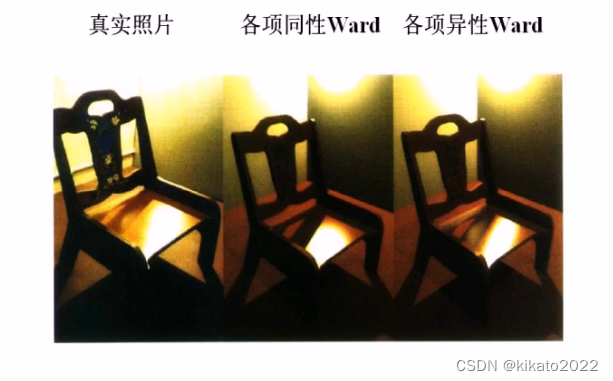###### 更多的BRDF类模型

Oren-Nayar模型[Generalization of Lambert’s Reflectance Model ACM SIGGRAPH, 1994]
- 将微平面当做 Lambertian 反射体而不是镜子
Poulin-Fournier 模型[ A Model for Anisotropic Reflection, ACM SIGGRAPH, 1990]
- 将微平面的法向表示为一组平行的圆柱体，同样处理各项异性的情况
波动光学（Wave optic）相关模型
- 由波动光学原理发展而来
- 材料的微平面大小和光的波长相当
- 基于不同的衍射（diffraction）理论，有两个工作[He, etal., A Comprehensive Physical Model for Light Reflection, ACM SIGGRAPH, 1991] 和 [Stam, Diffraction Shaders, ACM SIGGRAPH, 1999]
-虽然有很强的描述能力，但由于模型本身过于复杂而限制了它们的应用
由波动光学相关的模型得到的效果：##### 数据驱动的模型

度量一个大的材料集合的 BRDF，并将其记录为高维向量
利用降维方法从这些数据中计算一个低维流型
代表性工作：Matusik et al. [A Data-Driven Reflectance Model, ACM SIGGRAPH, 2003]

##### BRDF 模型表达形式的对比

经验模型（Empirical Models）：
- 计算简单，可以产生视觉上可接受的结果
物理模型（Physical-Based Models）：
- 参数具有物理意义
- 基于科学知识构建
数据驱动模型（Data-driven Models）：
- 灵活，对材料属性没有假设限定
- 很大的数据集，通常需要数据降维方法来压缩数据
- Needs interpolating data， missing high light parts

#### BRDF度量、评价

动机
- 为了对具有未知反射属性的材料进行建模，并生成具有高度真实感的结果
- 恢复 BRDF 和其它场景属性的过程有时也被称作逆渲染
- BRDF 度量概述
- 度量设备
- 实际获取中的问题
度量设备
- 由于 BRDF 是一个关于光线和视角的函数，其度量可通过对 2D 光照空间和2D视角空间进行采样获取。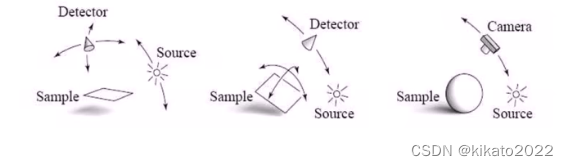测量设备：Light Stage
- Debevec et al. [SIGGRAPH 2000]
- 从固定物体（或人）上采集 BRDF
- 从少数的几个视角拍摄 2000 多个不同光源方向条件下的图像
- 从所有图像点上同时获取清楚的BRDF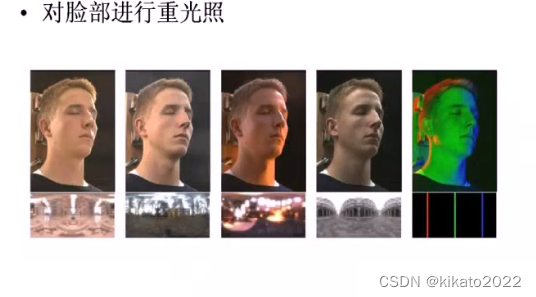测量设备：Gonioreflectometer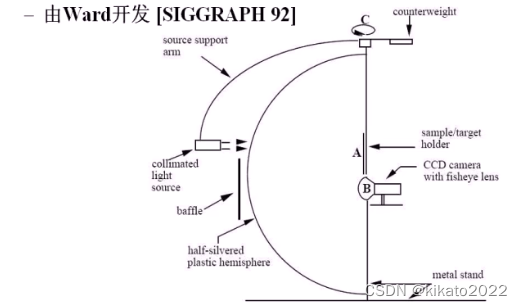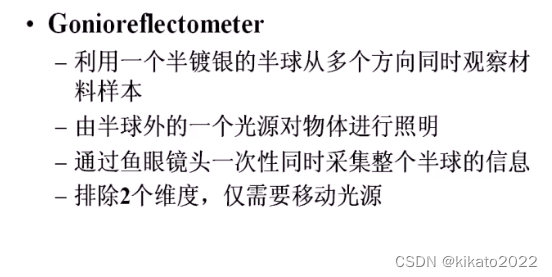展开全文算法
• 本文目录一、预备知识二、BRDF三、Phong光照模型 本文参考了本篇博客，同时加入了Phong光照模型 一、预备知识 在介绍光照模型和BRDF（双向反射分布函数）时，我们要先理解一些基本概念，这些概念与辐射度学有关 1. ...

### 本文目录

本文参考了本篇博客，同时加入了Phong光照模型

## 一、预备知识

在介绍光照模型和BRDF（双向反射分布函数）时，我们要先理解一些基本概念，这些概念与辐射度学有关

1. 能量

每个光子都具有一定的能量（Energy），能量大小与频率有关 E = h v E = hv 。用符号 Q Q 表示，单位是焦耳 J J

2. 功率

功率、辐射通量、通量

功率（Power），也被称为辐射通量（Radiant Flux）或者通量（Flux），指的是单位时间内通过表面或者空间区域的能量的总量。用符号 Φ \Phi 表示， Φ = d Q d t \Phi = \frac{dQ}{dt} ，单位为瓦特 W W ，或者焦耳/秒 J / S J/S

3. 辐照度和辐出度

辐照度（辐射通量密度）

辐出度、辐射出射度、辐射度（辐射通量密度）

• 辐照度（Irradiance）指单位时间内到达单位面积的辐射能量，或单位面积的辐射通量，即通量对于面积的密度。用符号 E E 表示， E = d Φ d A = d Q d t d A E = \frac{d\Phi}{dA} = \frac{dQ}{dtdA} ，单位 W / m 2 W/m^{2}

注意辐射通量和辐射通量密度之间的区别

如下图所示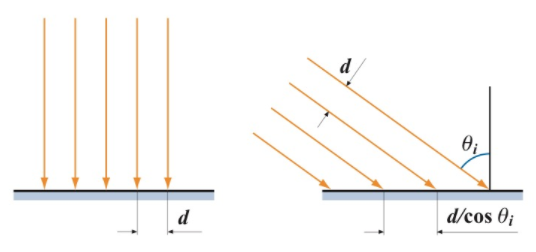一束平行光之间的间距为 d d ，一个垂直入射，一个与表面法线成 θ i \theta_{i} 的角度入射，观察表面的间距，其中左图间距为 d d ，右图为 d c o s θ i \frac{d}{cos\theta_{i}} ，相对于左图，右图的间距增大了。由于辐射通量是单位时间内通过表面或空间区域内的能量总和，所以辐射通量相同；而辐射通量密度是单位时间内通过单位面积的能量，右边面积大，所以右边辐射通量密度变小

让我们来看一下二者辐射通量密度的差异：假设右图中不垂直于光线方向的表面面积为 A A ，将他投影到垂直于直线方向得到一个虚拟表面，面积为 A ⊥ = A c o s θ i A^{\perp } = Acos\theta_{i} ，通过这两个面积的通量相同，设为 Φ \Phi ，则表面接收到的辐射通量密度 E = Φ A E = \frac{\Phi}{A} ，虚拟表面的辐射通量密度 E ⊥ = Φ A ⊥ = Φ A c o s θ i E_{\perp} = \frac{\Phi}{A^{\perp}} = \frac{\Phi}{Acos\theta_{i}} ，得到 E = E ⊥ c o s θ i E = E_{\perp}cos\theta_{i}

同理对于点光源，假想以点光源为中心不同半径的求包围着点光源，穿过这些球的辐射通量是相通的，均为 Φ \Phi ，球的表面积为 4 π r 2 4\pi r^{2} ，得到辐射通量密度 E = Φ 4 π r 2 E = \frac{\Phi}{4\pi r^{2}} ，即通量密度与距离的平方成反比，即光的衰减与距离的平方成反比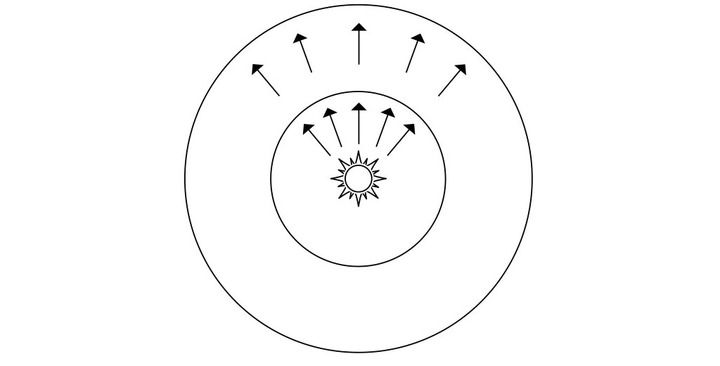4. 辐射强度

辐射强度

立体角：度量三维角度的量，用符号 ω \omega 表示，立体角计算为 ω = s r 2 \omega = \frac{s}{r^{2}} ，单位球的表面积为 4 π r 2 = 4 π 4\pi r^{2} = 4\pi ，所以球面的立体角也为 4 π 4\pi

辐射强度（Radiant Intensity），通过单位立体角的辐射能量，用符号 I I 表示， I = d Φ d w I=\frac{d\Phi}{dw} ，单位 W / s r W/sr

引入辐射强度的原因是：有时候需要度量通过一个点的通量的密度，因为点的面积是0，无法直接使用辐射通量密度，所以引入辐射强度。辐射强度不会随距离的增大而衰减，这是因为立体角不会随距离的变化而变化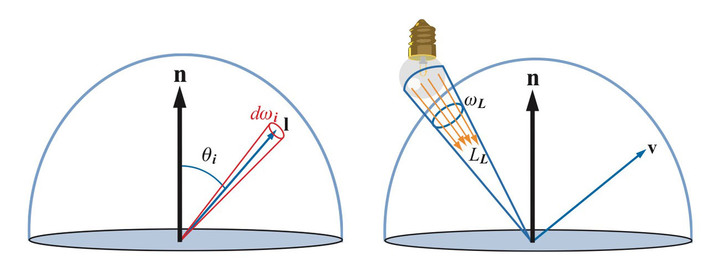5. 辐射率

辐射率

辐射率（Radiance），每单位面积每单位立体角的辐射通量密度，用符号 L L 表示， L = d Φ d ω d A ⊥ L = \frac{d\Phi}{d\omega dA^{\perp}} ，单位为 W / m 2 s r W/m^{2}sr ，其中 d A ⊥ dA^{\perp} 是微分面积 d A dA 在垂直于光线方向的投影，如图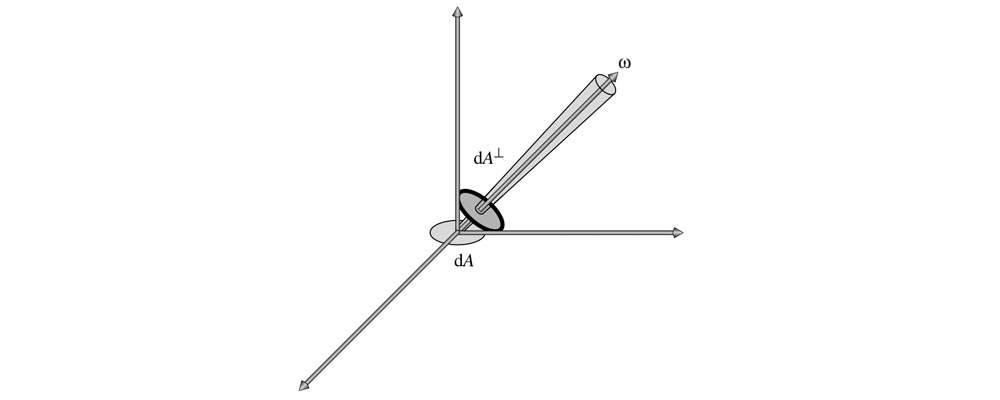辐射率可以看成我们眼睛看到（相机拍到）的物体上一点的颜色，基于物理着色时，计算表面一点的颜色就是计算它的辐射率，辐射率不会随距离变化而衰减，这和我们日常感受一致，在没有雾霾的干扰时，我们看到的物体表面上一点的颜色并不会随距离变化而变化。为什么辐照度会随距离增大而衰减，但是我们看到的颜色却不会衰减呢？这是因为随着距离变大，我们看到的物体上的一块区域到达视网膜的通量密度会变小，同时这块区域在视网膜表面上的立体角也会变小，正好抵消了通量密度的变化

## 二、BRDF

我们看到一个表面，实际上是周围环境的光照射到表面上，然后表面将一部分光反射到我们眼睛里。双向反射分布函数BRDF（Bidirectional Reflectance Distribution Function）就是描述表面入射光和反射光关系的

对于一个方向的入射光，表面会将光反射到表面上半球的各个方向，不同方向反射的比例是不同的，我们用BRDF来表示指定方向的反射光和入射光的比例关系，BRDF定义为：

f ( l , v ) = d L o ( v ) d E ( l ) f(l, v) = \frac{dL_{o}(v)}{dE(l)}

• f f ：BRDF， l l 是入射光方向， v v 是观察方向，即反射光方向
• d L o ( v ) dL_{o}(v) ：表面反射到 v v 方向的反射光的微分辐射率。表面反射到 v v 方向的反射光的辐射率为 L o ( v ) L_{o}(v) ，来自于表面上半球所有方向的入射光线的贡献，而微分辐射率 d L o ( v ) dL_{o}(v) 特指来自方向 l l 的入射光贡献的反射辐射率
• d E ( l ) dE(l) ：表面上来自入射光方向 l l 的微分辐照度。表面接收到的辐照度为 E E ，来自上半球所有方向的入射光线的贡献，而微分辐照度 d E ( l ) dE(l) 特指来自于方向 l l 的入射光

表面对不同频率的光反射率可能不一样，因此BRDF和光的频率有关。在图形学中，将BRDF表示为RGB向量，三个分量各有自己的 f f 函数

BRDF需要处理表面上半球的各个方向，如下图使用球坐标系定义方向更加方便。球坐标系使用两个角度来确定一个方向：

• 方向相对法线的角度 θ \theta ，称为极角（Polar Angle）或天顶角（Zenith Angle）
• 方向在平面上的投影相对于平面上一个坐标轴的角度 ϕ \phi ，称为方位角（Azimuthal Angle）

所以BRDF也可以表示成 f ( θ i , ϕ i , θ o , ϕ o ) f(\theta_i, \phi_i, \theta_o, \phi_o) 。对于各向同性材质，当 l l v v 同时绕法线 n n 旋转时， f f 值保持不变，此时可以用 l l v v 在平面投影的夹角 ϕ \phi 来代替 ϕ i \phi _i ϕ o ： f ( θ i , θ o , ϕ ) \phi _o：f(\theta_i, \theta_o, \phi)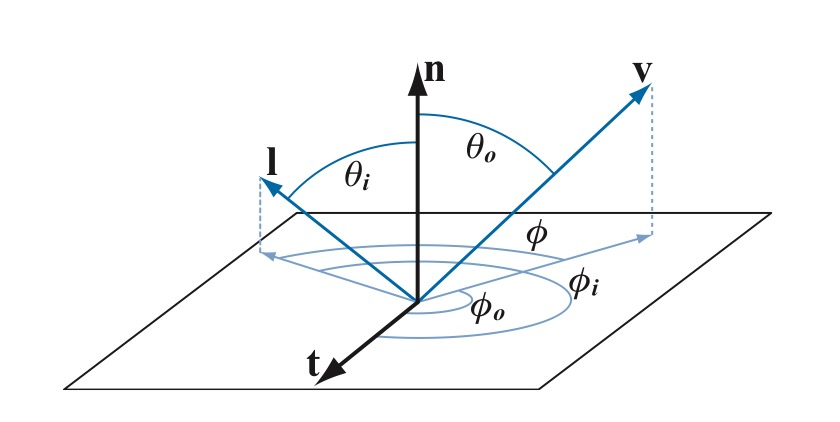为什么BRDF要定义成辐射率和辐照度的比值，而不是直接定义为辐射率和辐射率比值，有两种解释

第一种解释参考BRDF为什么要定义为一个单位是sr-1的量？

结合下面辐射通量密度 ( A ) (A) 和辐射率 ( B ) (B) 测量仪的示意图来看：辐照度(辐射通量密度)测量仪 ( A ) (A) 接受平面上半球的所有光线，可以测量一个较小面积来自于四面八方的所有光通量，光通量 Φ \Phi 除以传感器面积 A A 就可以得到辐照度(辐射通量密度) E E 。辐射度测量仪 ( B ) (B) 则有一个长筒控制光线只能从一个很小的立体角进入测量仪，光通量 Φ \Phi 除以传感器面积 A A 和立体角 ω \omega 就可以得到辐射率 L L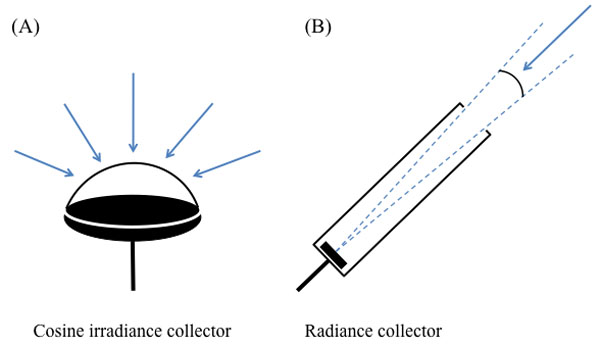测平面上一点在某一个方向的出射辐射率很简单，只需要用仪器 ( B ) (B) 从该方向对准该点就可以了。而测平面一点入射的辐射率则没有那么简单，必须保证光源正好覆盖测量仪开口立体角，大了该点会接受到比测量值更多的光照，导致测量值比实际值小，小了则与仪器的设计立体角不一致，可在实际中是基本做不到光源大小正好覆盖测量仪开口立体角的。而测表面的辐照度则简单得多，只要保证光源很小，而且没有来自其他方向的光干扰，这时候测到的辐照度就是平面上来自光源方向的微分辐照度 d E dE

第二种解释从数学的角度出发

对于现实世界中的非光学平面，一束光线射到表面上后，被表面反射到各个方向，其中一个出射方向的光通量只是整个反射光通量极小的一部分，当出射方向立体角趋于0时， lim ⁡ ω o → 0 d L o L i = 0 \lim_{\omega_{o} \rightarrow 0}{\frac{dL_{o} }{Li} } = 0 ，所以在实际计算中使用辐射率和辐射率比值是没有意义的。而如果分母改成表面上接收到的来自光源方向的微分辐照度，我们知道 d E = L i ( l ) d ω i c o s θ i dE = L_i(l) d\omega _{i} cos \theta _{i} ，由于给入射辐射率乘了一个趋于零的微分立体角， d E dE 的值会小很多，比值 d L o d E \frac{dL_o}{dE} 是有意义的，而不是0

下面我们来看看怎么用BRDF来计算表面辐射率

我们考虑来自方向 l l 的入射光辐射率 L i ( l ) L_i(l) ，由辐射率和辐照度的定义：

L i ( l ) = d Φ d ω i d A ⊥ = d Φ d ω i d A c o s θ i = d E ( l ) d ω i c o s θ i L_i(l) = \frac{d \Phi }{d\omega_i d A^{\bot } } = \frac{d \Phi }{d\omega_i dA cos \theta_i } = \frac{dE(l) }{d\omega_i cos \theta_i }

则照射到表面来自于方向l的入射光贡献的微分辐照度：

d E ( l ) = L i ( l ) d ω i c o s θ i dE(l) = L_i(l) d\omega_i cos \theta_i

表面反射到 v v 方向的由来自于方向l的入射光贡献的微分辐射率：

d L o ( v ) = f ( l , v ) ⊗ d E ( l ) = f ( l , v ) ⊗ L i ( l ) d ω i c o s θ i dL_o(v) = f(l, v) \otimes dE(l) = f(l, v) \otimes L_i(l) d\omega_i cos \theta_i
符号 ⊗ \otimes 表示按向量的分量相乘，因为 f f L i L_i 都包含RGB三个分量

要计算表面反射到v方向的来自上半球所有方向入射光线贡献的辐射率，可以将上式对半球所有方向的光线积分：

L o ( v ) = ∫ Ω f ( l , v ) ⊗ L i ( l ) c o s θ i d ω i L_o(v) = \int_{\Omega }^{} f(l, v) \otimes L_i(l) cos \theta_i d\omega_i
上式称为反射方程（Reflectance Equation），用来计算表面反射辐射率

对于点光源、方向光等理想化的精准光源（Punctual Light），计算过程可以大大简化。我们考察单个精准光源照射表面，此时表面上的一点只会被来自一个方向的一条光线照射到（而面积光源照射表面时，表面上一点会被来自多个方向的多条光线照射到），则辐射率：

L o ( v ) = f ( l , v ) ⊗ E L c o s θ i L_o(v) = f(l, v) \otimes E_L cos \theta_i
对于多个精准光源，只需简单累加就可以了：

L o ( v ) = ∑ k = 1 n f ( l k , v ) ⊗ E L k c o s θ i k L_o(v) = \sum_{k = 1}^{n}{f(l_k, v) \otimes E_{L_k} cos \theta_{i_k}}
这里使用光源的辐照度，对于阳光等全局方向光，可以认为整个场景的辐照度是一个常数，对于点光源，辐照度随距离的平方衰减，用公式 E L = Φ 4 π r 2 E_{L} = \frac{\Phi }{4\pi r^2} 就可以求出到达表面的辐照度， Φ \Phi 是光源的功率，比如100瓦的灯泡，r是表面离光源的距离

回头看看反射方程，是对表面上半球所有方向的入射光线积分，这里面包含了来自精准光源的光线，也包括周围环境反射的光线。处理来自周围环境的光线可以大幅提高光照的真实程度，在实时图形学中，这部分光照可以用基于图像的光照（Image Based Lighting）来模拟。我们将在下篇文章讨论基于图像的光照。

上面给出了BRDF的定义和使用BRDF计算表面反射辐射率的公式。但这个定义实际上是无法直接用于计算表面反射辐射率的，我们还要建立一个能模拟真实光照的模型，使得输入入射方向和出射方向， f ( l , v ) f(l, v) 能输出表面反射微分辐射率和入射微分辐照度的比率。

1967年Torrance-Sparrow在Theory for Off-Specular Reflection From Roughened Surfaces中使用辐射度学和微表面理论建立了模拟真实光照的BRDF模型，1981年Cook-Torrance在A Reflectance Model for Computer Graphics中把这个模型引入到计算机图形学领域，现在这个模型已经成为基于物理着色的标准，被称为Cook-Torrance模型。下面我们来看看微表面理论和Cook-Torrance模型的推导过程。

## 三、Phong光照模型

光照到物体表面时，物体对光会发生反射、透射和吸收。简单光照模型只考虑物体对直接光照的反射作用，而物体间的光反射作用，只用环境光来表示。此时光源被假定为点光源，反射作用被细分为镜面反射和漫反射

Phong光照模型

根据Phong光照模型，物体被感知的亮度由环境光、漫反射光及镜面反射光组成，物体表面的反射光强度为
I = K a I a + K d I l c o s ( θ ) + K s I l c o s n ( α ) I = K_{a}I_{a} + K_{d}I_{l}cos(\theta) + K_{s}I_{l}cos^{n}(\alpha)

• K a   K d   K s K_{a}~K_{d}~K_{s} ：分别为环境光、漫反射光及镜面反射光系数
• I a I_{a} ：入射的环境光强度
• I l I_{l} ：光源的入射光强度
• θ \theta ：入射光与物体表面法线之间的夹角
• α \alpha ：反射光与视线之间的夹角
• n n ：镜面反射参数

1. 漫反射

理想漫反射当光源来自一个方向时，漫反射光均匀向各方向传播，与视点无关，他是由表面的粗糙不平引起的，因而漫反射光的空间分布是均匀的。记入射光强为 I p I_{p} ，物体表面上点 P P 的法向量为 N N ，从点 P P 指向光源的向量为 L L ，当 L 、 N L、N 为单位向量时，理想漫反射光强表示为：
I d = I p K d ( L ⋅ N ) I_{d} = I_{p}K_{d}(L\cdot N)

K d K_{d} ：与物体有关的漫反射系数，取值 ( 0 , 1 ) (0, 1) 。在RGB模型下，漫反射系数 K d K_{d} 有三个分量 K d r 、 K d g 、 K d b K_{dr}、K_{dg}、K_{db} 分别表示RGB三原色的漫反射系数，反应物体颜色，通过调整三个分量可以改变物体的颜色，也可以吧入射光强 I I 设为三个分量 I r 、 I g 、 I b I_{r}、I_{g}、I_{b} 通过调整这些分量来调整光源的颜色

2. 镜面反射

镜面反射光对于理想镜面，反射光集中在一个方向，并遵守反射定律。对一般光滑表面，反射光集中在一个范围内，且由反射定律决定的反射方向光强最大。因此对于同一点来说，从不同位置所观察到的镜面反射光强是不同的，镜面反射光强可以表示为：
I s = I p K s c o s n α     α ∈ ( 0 , π 2 ) I_{s} = I_{p}K_{s}cos^{n}\alpha~~~\alpha \in (0, \frac{\pi}{2})

• K s K_{s} ：与物体有关的镜面反射系数
• α \alpha ：视线方向 V V 与反射方向 R R 的夹角
• n n ：反射指数，反映了物体表面的光泽程度，一般为1~2000，数目越大表示物体表面越光滑

镜面反射光将会在反射方向附近形成很亮的光斑，称为高光现象，将 V 和 R V和R 看做单位向量，镜面反射光强可以重写为：
I s = I p K s ( R ⋅ V ) n I_{s} = I_{p}K_{s}(R\cdot V)^{n}

其中 R = 2 N ( N ⋅ L ) − L R = 2N(N\cdot L)-L ，镜面反射光产生的高光区域只反映光源的颜色，例如在红光照射下，一个物体的高光域是红光，镜面反射系数 K s K_{s} 是一个与物体颜色无关的参数，在简单光照明模型中，只能通过改变物体的漫反射系数来控制物体的颜色

3. 环境光

环境光指光源间接对物体的影响，是在物体和环境之间多次反射，最终达到平衡时的一种光，可以近似地认为同一环境下的环境光，其光强分布是均匀的，他在任何一个方向上的分布都相同，在简单光照模型中，用一个常数来模拟环境光，用式子表示为：
I e = I a K a I_{e} = I_{a}K_{a}

展开全文• BRDF理论及shader实现（上） Specular BRDF 对于specular分量来说，fmf_mfm​是一个遵循菲涅尔反射定律的镜面BRDF项，此时的fmf_mfm​满足（和有详细的推导）： fm(l,v,m)=F(v,m)δωm(h,m)4(l⋅h)2 f_m({\bf...

## Specular BRDF

对于specular分量来说， f m f_m 是一个遵循菲涅尔反射定律的镜面BRDF项，此时的 f m f_m 满足（和有详细的推导）：

f m ( l , v , m ) = F ( v , m ) δ ω m ( h , m ) 4 ( l ⋅ h ) 2 f_m({\bf{l}},{\bf{v}},{\bf{m}}) = F({\bf{v}},{\bf{m}})\frac{\delta_{\omega_m}({\bf{h}}, {\bf{m}})}{4({\bf{l}}\cdot{\bf{h}})^2}

h {\bf{h}} 表示half vector，是 v {\bf{v}} l {\bf{l}} 的平均；这里分母上第一次出现了 4 4 ，这也是后面specular BRDF公式的分母上的 4 4 的来源。 δ ω m ( s , o ) \delta_{\omega_m}({\bf{s}}, {\bf{o}}) 记为：

∫ Ω g ( o ) δ ω o ( s , o ) d ω o = { g ( s ) , if  s ∈ Ω 0 , otherwise \int_\Omega g({\bf{o}})\delta_{\omega_o}({\bf{s}}, {\bf{o}})d\omega_o = \begin{cases} g({\bf{s}}), & \text {if $s\in\Omega$} \\ 0, & \text{otherwise} \end{cases}

此时， f r f_r 可以化简为为：

f r ( l , v ) = D ( h , α ) G ( v , l , α ) F ( v , h , F 0 ) 4 ( n ⋅ v ) ( n ⋅ l ) f_r({\bf{l}},{\bf{v}}) = \frac{D({\bf{h}},\alpha)G({\bf{v}},{\bf{l}},\alpha)F({\bf{v}},{\bf{h}},F_0)}{4({\bf{n}}\cdot{\bf{v}})({\bf{n}}\cdot{\bf{l}})}

其中， α \alpha 取决于我们前面提到的粗糙度roughness，具体为

α = r o u g h n e s s 2 \alpha = roughness^2

可以理解为 α \alpha 是对粗糙度roughness的一个映射， α \alpha 将多次被用到。

菲涅尔项及 F 0 F_0 会在后面详细介绍，这里暂时略过。

// #define saturate(x) clamp(x, 0, 1)
// N     = normal;
// V     = normalize(camPos - WorldPos);
// L     = normalize(LightPos - WorldPos));
// H     = normalize(V + L);
// NdotV = saturate(dot(N, V));
// NdotL = saturate(dot(N, L));
// NdotH = saturate(dot(N, H));
// LdotH = saturate(dot(L, H));
// VdotH = saturate(dot(V, H));
vec3 SpecularBRDF(float NdotV, float NdotL, float NdotH, float LdotH, float VdotH, vec3 F0, float roughness) {
float r   = roughness * roughness;

float D = Distribution(NdotH, r);
float V = Geometry(NdotV, NdotL, r);
vec3  F = Fresnel(VdotH, F0);

return D * V * F / (4 * NdotV * NdotL);
}


注意，这里的NdotLNdotV等都是clamp到0到1的。

接下来具体看 f r f_r 中每个分量的可能形式都有哪些。

### 法向分布函数 D

这一部分介绍3个法向分布函数的公式，以及一个推广。

#### Beckmann

来源， D B e c k m a n n D_{Beckmann} 假设微表面的法向分布是以 n {\bf{n}} 为均值的高斯分布，也即 h {\bf{h}} n {\bf{n}} 越接近，反射的光线越多， D B e c k m a n n D_{Beckmann} 越大。再结合 D B e c k m a n n D_{Beckmann} 的积分约束，求得：

D B e c k m a n n ( h , α ) = χ + ( n , h ) π α 2 ( n ⋅ h ) 4 e ( n ⋅ h ) 2 − 1 α 2 ( n ⋅ h ) 2 D_{Beckmann}({\bf{h}}, \alpha) = \frac{\chi^+({\bf{n}},{\bf{h}})}{\pi\alpha^2({\bf{n}}\cdot{\bf{h}})^4}e^{\frac{({\bf{n}}\cdot{\bf{h}})^2-1}{\alpha^2({\bf{n}}\cdot{\bf{h}})^2}}

这个公式有一个很致命的缺陷，那就是当roughness接近于1的时候， D B e c k m a n n D_{Beckmann} n ⋅ h ∈ [ 0 , 1 ] {\bf{n}}\cdot{\bf{h}}\in[0,1] 区间内，不是单调递增的。表现在渲染上，就是在高光最强的中心点会产生一个暗斑。float DistributionBeckmann(float NdotH, float r) {
float NdotH2   = NdotH * NdotH;
float r2       = r * r;
float r2NdotH2 = r2 * NdotH2;
return exp((NdotH2 - 1) / (r2NdotH2)) / (PI * r2NdotH2 * NdotH2);
}


#### BlinnPhong

来源，BlinnPhong公式纯粹是基于经验的，在恰当选取参数的情况下，它的函数曲线非常接近于Beckmann。

BlinnPhong原始的模型是：

D B l i n n ( h , α ) = χ + ( n , h ) α p + 2 2 π ( n ⋅ h ) α p D_{Blinn}({\bf{h}}, \alpha) = \chi^+({\bf{n}},{\bf{h}})\frac{\alpha_p + 2}{2\pi}({\bf{n}}\cdot{\bf{h}})^{\alpha_p}

其中， α p \alpha_p 表示粗糙系数，或者准确的说，是光滑系数—— α p \alpha_p 越大，表示物体表面越光滑。

• α p = ∞ \alpha_p=\infty 的时候，表示绝对光滑的物体，此时 D B l i n n ( h , α ) D_{Blinn}({\bf{h}}, \alpha) 只有在 h = n {\bf{h}} = {\bf{n}} ，即入射角等于出射角的时候为 ∞ \infty ，否则为0。
• α p = 0 \alpha_p=0 的时候，表示绝对粗糙的物体， D B l i n n ( h , α ) = 1 π D_{Blinn}({\bf{h}}, \alpha) = \frac{1}{\pi} ，这个式子也是后面会提到的diffuse的式子。

α p = ( 2 α 2 − 2 ) \alpha_p = (\frac{2}{\alpha^2} - 2) ，则有：

D B l i n n ( h , α ) = χ + ( n , h ) π α 2 ( n ⋅ h ) ( 2 α 2 − 2 ) D_{Blinn}({\bf{h}}, \alpha) = \frac{\chi^+({\bf{n}},{\bf{h}})}{\pi\alpha^2}({\bf{n}}\cdot{\bf{h}})^{(\frac{2}{\alpha^2} - 2)}

这个公式即接近于Beckmann的法向分布公式，也是常用的BlinnPhong形式。

float DistributionBlinnPhong(float NdotH, float r) {
float a = r * r;
return pow(NdotH, 2.0 / a - 2.0) / (PI * a);
}


#### GGX

来源，GGX是根据实测数据拟合出来的一个公式：

D G G X ( h , α ) = χ + ( n , h ) ⋅ α 2 π ( ( n ⋅ h ) 2 ( α 2 − 1 ) + 1 ) 2 D_{GGX}({\bf{h}}, \alpha) = \frac{\chi^+({\bf{n}},{\bf{h}})\cdot\alpha^2}{\pi(({\bf{n}}\cdot{\bf{h}})^2(\alpha^2-1)+1)^2}

float DistributionGGX(float NdotH, float r) {
float a2     = r * r;
float NdotH2 = NdotH * NdotH;
float nom    = a2;
float denom  = (NdotH2 * (a2 - 1.0) + 1.0);
denom        = PI * denom * denom;
return nom / max(denom, 0.001);
}


除了这三种公式，还有更多更复杂的法向分布函数D，具体可以参考。但是其实最常用的还是GGX（及其各向异性模式），无论是游戏还是影视行业都比较喜欢用GGX。

#### GTR

Burley通过对Berry（与GGX公式类似，分母上的指数为1）和GGX公式的观察，提出了广义的Trowbridge-Reitz（Generalized-Trowbridge-Reitz，GTR）法线分布函数：

D G T R ( h , α ) = c ⋅ χ + ( n , h ) ( ( n ⋅ h ) 2 ( α 2 − 1 ) + 1 ) γ D_{GTR}({\bf{h}}, \alpha) = \frac{c\cdot\chi^+({\bf{n}},{\bf{h}})}{(({\bf{n}}\cdot{\bf{h}})^2(\alpha^2-1)+1)^\gamma}

其中， c c 表示缩放系数，是一个常数； γ \gamma 用于控制尾部的形状，当 γ = 1 \gamma=1 的时候， D G T R D_{GTR} 就是Berry公式，当 γ = 2 \gamma=2 的时候， D G T R D_{GTR} 就是 D G G X D_{GGX}

γ \gamma 的取值对 D G T R D_{GTR} 的影响如下图所示。以下是 γ = 1 \gamma=1 γ = 2 \gamma=2 时的shader实现：

float DistributionGTR1(float NdotH, float r)
{
if (r >= 1) return 1/PI;
float a2 = r*r;
float t = 1 + (a2-1)*NdotH*NdotH;
return (a2-1) / (PI*log(a2)*t);
}

float DistributionGTR2(float NdotH, float r)
{
float a2 = r*r;
float t = 1 + (a2-1)*NdotH*NdotH;
return a2 / (PI * t * t);
}


#### 效果对比可以看出，BlinnPhong和Beckmann的差异不大。而GGX有着更平滑的边缘和更小的峰值。除此之外，GGX运算压力更小，因为它没有指数操作。

### 遮挡项 G

和法向分布函数 D D 一样，遮挡项 G G 也是入射角、出射角和表面粗糙度的函数。

有些文章会把遮挡项G和BRDF的分母 ( n ⋅ l ) ( n ⋅ v ) ({\bf{n}}\cdot{\bf{l}})({\bf{n}}\cdot{\bf{v}}) 放在一起组成一项约分掉，这也是一种优化思路，因为G通常包含这两个cosine因子。这里我们约定本文的遮挡项 G G 是不约分 ( n ⋅ l ) ( n ⋅ v ) ({\bf{n}}\cdot{\bf{l}})({\bf{n}}\cdot{\bf{v}}) G G

#### Implicit

来源，有些BRDF公式会忽略遮挡项G，将其跟分母上的 ( n ⋅ l ) ( n ⋅ v ) ({\bf{n}}\cdot{\bf{l}})({\bf{n}}\cdot{\bf{v}}) 一起忽略掉，这就有了第一个隐式 G G

G I m p l i c i t ( l , v , h ) = ( n ⋅ l ) ( n ⋅ v ) G_{Implicit}({\bf{l}},{\bf{v}},{\bf{h}})=({\bf{n}}\cdot{\bf{l}})({\bf{n}}\cdot{\bf{v}})

它的形态大概是，当且仅当视角和光源都垂直于物体表面的时候， G I m p l i c i t = 1 G_{Implicit}=1 ，光源、视角与物体表面法线的夹角越大， G I m p l i c i t G_{Implicit} 越小，直到衰减为0，这也是很符合常识的。

float GeometryImplicit(float NdotV, float NdotL) {
return NdotL * NdotV;
}


但是隐式遮挡项 G I m p l i c i t G_{Implicit} 最大的问题在于，它随着视角的衰减速度太快，这会使得高光区域太窄。为了解决这个问题，我们继续看显式的遮挡项 G G

#### Cook-Torrance

来源， G C o o k − T o r r a n c e G_{Cook-Torrance} 解决了 G I m p l i c i t G_{Implicit} 衰减速度太快的问题：

G C o o k − T o r r a n c e ( l , v , h ) = min ⁡ ( 1 , 2 ( n ⋅ h ) ( n ⋅ v ) v ⋅ h , 2 ( n ⋅ h ) ( n ⋅ l ) v ⋅ h ) G_{Cook-Torrance}({\bf{l}},{\bf{v}},{\bf{h}})=\min{\left(1, \frac{2({\bf{n}}\cdot{\bf{h}})({\bf{n}}\cdot{\bf{v}})}{{\bf{v}}\cdot{\bf{h}}}, \frac{2({\bf{n}}\cdot{\bf{h}})({\bf{n}}\cdot{\bf{l}})}{{\bf{v}}\cdot{\bf{h}}}\right)}

float GeometryCookTorrance(float NdotV, float NdotL, float VdotH, float NdotH) {
float ct1 = 2 * NdotH * NdotV / VdotH;
float ct2 = 2 * NdotH * NdotL / VdotH;
return min(1, min(ct1, ct2));
}


#### Kelemen

来源，也是解决 G I m p l i c i t G_{Implicit} 衰减速度太快的问题，同时 G K e l e m e n G_{Kelemen} G C o o k − T o r r a n c e G_{Cook-Torrance} 的效率更高：

G K e l e m e n ( l , v , h ) = ( n ⋅ l ) ( n ⋅ v ) ( v ⋅ h ) 2 G_{Kelemen}({\bf{l}},{\bf{v}},{\bf{h}})=\frac{({\bf{n}}\cdot{\bf{l}})({\bf{n}}\cdot{\bf{v}})}{({\bf{v}}\cdot{\bf{h}})^2}

float GeometryKelemen(float NdotV, float NdotL, float VdotH) {
return NdotV * NdotL / (VdotH * VdotH);
}


#### Neumann

来源， G N e u m a n n G_{Neumann} 用另一种方式解决了 G I m p l i c i t G_{Implicit} 衰减速度太快的问题：

G N e u m a n n ( l , v , h ) = ( n ⋅ l ) ( n ⋅ v ) max ⁡ ( n ⋅ l , n ⋅ v ) G_{Neumann}({\bf{l}},{\bf{v}},{\bf{h}})=\frac{({\bf{n}}\cdot{\bf{l}})({\bf{n}}\cdot{\bf{v}})}{\max{({\bf{n}}\cdot{\bf{l}}, {\bf{n}}\cdot{\bf{v}}})}

float GeometryNeumann(float NdotV, float NdotL) {
return (NdotL * NdotV) / max(NdotL, NdotV);
}


但是，以上三个解决方案也不够完美。前面提到过，遮挡项G应该是入射角、出射角和表面粗糙度的函数，而以上四个G，包括隐式遮挡项都与粗糙度无关。

#### Smith

Smith家族都是采用了前面介绍的 G 1 G_1 相乘的形式：

G 2 ( l , v , h ) = G 1 ( l ) G 1 ( v ) G_2({\bf{l}},{\bf{v}},{\bf{h}})=G_1({\bf{l}})G_1({\bf{v}})

他们之间的区别就是 G 1 G_1 的选取不同。

##### Beckmann

Beckmann的 G G 是跟 D D 一起提出的，前面介绍过 G G 是可以从 D D 推导出来的，因此Beckmann的 Λ \Lambda 为：

c = n ⋅ v α 1 − ( n ⋅ v ) 2 Λ ( v ) = erf ( c ) − 1 2 + 1 2 c π exp ⁡ ( − c 2 ) \begin{aligned} c & = \frac{{\bf{n}}\cdot{\bf{v}}}{\alpha\sqrt{1-({\bf{n}}\cdot{\bf{v}})^2}} \\ \Lambda({\bf{v}}) & = \frac{\text{erf}(c)-1}{2}+\frac{1}{2c\sqrt{\pi}}\exp(-c^2) \end{aligned}

但是由于有 erf \text{erf} 函数的存在，计算起来过于复杂，因此通常用如下的近似形式：

Λ ( v ) ≈ { 1 − 1.259 x + 0.396 c 2 3.535 c + 2.181 c 2 , if  c < 1.6 0 , if  c ≥ 1.6 \Lambda({\bf{v}}) \approx \begin{cases} \frac{1-1.259x+0.396c^2}{3.535c+2.181c^2}, & \text{if }c<1.6 \\ 0, & \text{if }c\geq1.6 \end{cases}

因此，Beckmann的 G 1 G_1

G B e c k m a n n ( v ) ≈ { 3.535 c + 2.181 c 2 1 + 2.276 c + 2.577 c 2 , if  c < 1.6 1 , if  c ≥ 1.6 G_{Beckmann}({\bf{v}}) \approx \begin{cases} \frac{3.535c+2.181c^2}{1+2.276c+2.577c^2}, & \text{if }c<1.6 \\ 1, & \text{if }c\geq1.6 \end{cases}

float GeometryBeckmann(float NdotV, float r) {
float c  = NdotV / (r * sqrt(1 - NdotV * NdotV));
float c2 = c * c;
if (c < 1.6)
return (3.535 * c + 2.181 * c2) / (1 + 2.276 * c + 2.577 * c2);
else
return 1.0;
}
float GeometrySmithBeckmann(float NdotV, float NdotL, float r) {
float ggx2 = GeometryBeckmann(NdotV, r);
float ggx1 = GeometryBeckmann(NdotL, r);
return ggx1 * ggx2;
}

##### GGX

GGX跟Beckmann类似，都是从法向分布函数推导出来的：

c = n ⋅ v α 1 − ( n ⋅ v ) 2 Λ ( v ) = − 1 + 1 + 1 c 2 2 \begin{aligned} c & = \frac{{\bf{n}}\cdot{\bf{v}}}{\alpha\sqrt{1-({\bf{n}}\cdot{\bf{v}})^2}} \\ \Lambda({\bf{v}}) & = \frac{-1+\sqrt{1+\frac{1}{c^2}}}{2} \end{aligned}

对应的 G 1 G_1 定义为

G G G X ( v ) = 2 ( n ⋅ v ) ( n ⋅ v ) + α 2 + ( 1 − α 2 ) ( n ⋅ v ) 2 G_{GGX}({\bf{v}}) = \frac{2({\bf{n}}\cdot{\bf{v}})}{({\bf{n}}\cdot{\bf{v}})+\sqrt{\alpha^2+(1-\alpha^2)({\bf{n}}\cdot{\bf{v}})^2}}

float GeometryGGX(float NdotV, float r) {
float r2 = r * r;
return (2 * NdotV) / (NdotV + sqrt(r2 + (1 - r2) * NdotV * NdotV));
}
float GeometrySmithGGX(float NdotV, float NdotL, float r) {
float ggx2 = GeometryGGX(NdotV, r);
float ggx1 = GeometryGGX(NdotL, r);
return ggx1 * ggx2;
}

##### GGX Joint

前面提到的GGX用的是 G 2 = G 1 ∗ G 1 G_2=G_1*G_1 的separable G，如果用height-correlated G，那么 G 2 G_2 变为：

G 2 − G G X J o i n t ( l , v , m ) = 1 1 + Λ ( l ) + Λ ( v ) = 2 ( n ⋅ v ) ( n ⋅ l ) ( n ⋅ l ) ⋅ α 2 + ( 1 − α 2 ) ( n ⋅ v ) 2 + ( n ⋅ v ) ⋅ α 2 + ( 1 − α 2 ) ( n ⋅ l ) 2 \begin{aligned} G_{2-GGXJoint}({\bf{l}},{\bf{v}},{\bf{m}}) & =\frac{1}{1+\Lambda({\bf{l}})+\Lambda({\bf{v}})}\\ & =\frac{2({\bf{n}}\cdot{\bf{v}})({\bf{n}}\cdot{\bf{l}})}{({\bf{n}}\cdot{\bf{l}})\cdot\sqrt{\alpha^2+(1-\alpha^2)({\bf{n}}\cdot{\bf{v}})^2} + ({\bf{n}}\cdot{\bf{v}})\cdot\sqrt{\alpha^2+(1-\alpha^2)({\bf{n}}\cdot{\bf{l}})^2}} \end{aligned}

float GeometrySmithGGXJoint(float NdotV, float NdotL, float r) {
float r2 = r * r;
float Vis_SmithV = NdotL * sqrt(NdotV * (NdotV - NdotV * r2) + r2);
float Vis_SmithL = NdotV * sqrt(NdotL * (NdotL - NdotL * r2) + r2);
return 2 * NdotV * NdotL / (Vis_SmithV + Vis_SmithL);
}


为了提高计算效率，UE4对GGX Joint方法做了一个近似，公式为：

G 2 − G G X J o i n t ( l , v , m ) = 1 1 + Λ ( l ) + Λ ( v ) ≈ 2 ( n ⋅ v ) ( n ⋅ l ) ( n ⋅ l ) ⋅ ( α + ( 1 − α ) ( n ⋅ v ) ) + ( n ⋅ v ) ⋅ ( α + ( 1 − α ) ( n ⋅ l ) ) \begin{aligned} G_{2-GGXJoint}({\bf{l}},{\bf{v}},{\bf{m}}) & =\frac{1}{1+\Lambda({\bf{l}})+\Lambda({\bf{v}})}\\ & \approx\frac{2({\bf{n}}\cdot{\bf{v}})({\bf{n}}\cdot{\bf{l}})}{({\bf{n}}\cdot{\bf{l}})\cdot(\alpha+(1-\alpha)({\bf{n}}\cdot{\bf{v}})) + ({\bf{n}}\cdot{\bf{v}})\cdot(\alpha+(1-\alpha)({\bf{n}}\cdot{\bf{l}}))} \end{aligned}

float GeometryGGXJointApprox(float NdotV, float NdotL, float r) {
return (NdotV) / (NdotL * (r + (1 - r) * NdotV));
}
float GeometrySmithGGXJointApprox(float NdotV, float NdotL, float r) {
float Vis_SmithV = NdotL * ( NdotV * ( 1 - r ) + r );
float Vis_SmithL = NdotV * ( NdotL * ( 1 - r ) + r );
return 2 * NdotV * NdotL / ( Vis_SmithV + Vis_SmithL );
}

##### Schlick-Beckmann

Schlick的 G 1 G_1 定义为
k = α 2 π G S c h l i c k ( v ) = n ⋅ v ( n ⋅ v ) ( 1 − k ) + k k=\alpha\sqrt{\frac{2}{\pi}} \\ G_{Schlick}({\bf{v}})=\frac{{\bf{n}}\cdot{\bf{v}}}{({\bf{n}}\cdot{\bf{v}})(1-k)+k}

float GeometrySchlickBeckmann(float NdotV, float r) {
float k     = (r)*sqrt(2.0 / PI);
float nom   = NdotV;
float denom = NdotV * (1.0 - k) + k;
return nom / denom;
}
float GeometrySmithSchlickBeckmann(float NdotV, float NdotL, float r) {
float ggx2 = GeometrySchlickBeckmann(NdotV, r);
float ggx1 = GeometrySchlickBeckmann(NdotL, r);
return ggx1 * ggx2;
}

##### Schlick-GGX

Schlick-GGX曾经是UE4所采用的的一个模型，跟Schlick有些类似， G 1 G_1 定义为
k = α 2 G S c h l i c k ( v ) = n ⋅ v ( n ⋅ v ) ( 1 − k ) + k k=\frac{\alpha}{2} \\ G_{Schlick}({\bf{v}})=\frac{{\bf{n}}\cdot{\bf{v}}}{({\bf{n}}\cdot{\bf{v}})(1-k)+k}

float GeometrySchlickGGX(float NdotV, float r) {
float k     = r * 0.5;
float nom   = NdotV;
float denom = NdotV * (1.0 - k) + k;
return nom / denom;
}
float GeometrySmithSchlickGGX(float NdotV, float NdotL, float r) {
float ggx2 = GeometrySchlickGGX(NdotV, r);
float ggx1 = GeometrySchlickGGX(NdotL, r);
return ggx1 * ggx2;
}


这里面还有一个细节，那就是迪士尼后来提出了对粗糙粗roughness做一个remapping，使得它更接近于真实：
α ′ = ( r o u g h n e s s + 1 2 ) 2 \alpha' = (\frac{roughness + 1}{2})^2 \\

float GeometrySmithSchlickGGX(float NdotV, float NdotL, float roughness) {
float r = (roughness + 1.0) * 0.5; // remapping roughness
r = r * r
float ggx2 = GeometrySchlickGGX(NdotV, r);
float ggx1 = GeometrySchlickGGX(NdotL, r);
return ggx1 * ggx2;
}


注意，此时GeometrySmithSchlickGGX的输入参数不是r，而改为了roughness

#### 优化

考虑到几乎所有 G G 都带有 ( n ⋅ v ) ( n ⋅ l ) ({\bf{n}}\cdot{\bf{v}})({\bf{n}}\cdot{\bf{l}}) 项，可以跟 f r ( l , v ) f_r({\bf{l}},{\bf{v}}) 的分母约分，因此在实现时，可以考虑定义
G ′ = G ( n ⋅ v ) ( n ⋅ l ) G'=\frac{G}{({\bf{n}}\cdot{\bf{v}})({\bf{n}}\cdot{\bf{l}})}
节省一部分计算。

这样做不只是出于性能的考虑，也是出于精度的考虑。如果 n ⋅ v {\bf{n}}\cdot{\bf{v}} n ⋅ l {\bf{n}}\cdot{\bf{l}} 的乘积接近于0，那么specular项的分母会非常小，严重影响其精度，极端的情况下会在过渡区域产生一道割裂的分界线。下图展示了 ( n ⋅ v ) ∗ ( n ⋅ l ) ∗ 10 ({\bf{n}}\cdot{\bf{v}})*({\bf{n}}\cdot{\bf{l}})*10 （左）、未优化时（中）、优化后（右）的效果，可以看出左侧两张图的分界线非常吻合。优化后则没有颜色割裂的问题。#### 效果对比

roughness = 0.9，计算球体的遮挡项效果为：最后一排是常用的几种方法，差异并不大，边缘的过渡也比较好。

### 菲涅尔项 F

菲涅尔项描述的是物体表面的反射、折射现象。一般我们会采用常量 F 0 F_0 来计算菲涅尔项 F ( v , h , F 0 ) F({\bf{v}},{\bf{h}},F_0)

要说明白菲涅尔项，得从光学在介质表面的折射反射现象说起。我们知道光线会在介质表面产生不连续性，具体表现为一部分光线反射——遵循光线反射定律，入射角等于反射角；另一部分光线会折射进入介质——遵循光线折射定律，折射角取决于入射角的大小以及构成交界面的两种材质，即斯涅耳定律（Snell’s law）：

n 1 sin ⁡ ( θ i ) = n 2 sin ⁡ ( θ t ) n_1\sin(\theta_i)=n_2\sin(\theta_t)

斯涅耳定律描述的仅仅是光线的角度，但是图形学研究的其实是光线的radiance/irradiance，所以我们要更进一步。定义Fresnel reflectance R F R_F 为反射光线的radiance占入射光线radiance的比例， R F R_F 是入射角 θ i \theta_i 的函数。那么对于入射光线 L i L_i ，在角度 θ i \theta_i 时反射光线的radiance为 R F ( θ i ) L i R_F(\theta_i)L_i 。再考虑折射部分，根据能量守恒，没有反射的能量都会被折射（不考虑被吸收的能量），因此折射的flux占入射flux的比例是 1 − R F 1-R_F 。这里需要强调的是，radiance定义的是“irradiance每立体角”，它的大小跟角度有关系，因此折射光线的radiance L t L_t 不能简单用 1 − R F 1-R_F 乘上 L i L_i ，而要转换角度：
L t = ( 1 − R F ( θ i ) ) sin ⁡ 2 θ i sin ⁡ 2 θ t L i L_t = (1-R_F(\theta_i))\frac{\sin^2\theta_i}{\sin^2\theta_t}L_i
将斯涅耳定律带入上式，得到：
L t = ( 1 − R F ( θ i ) ) n 2 2 n 1 2 L i L_t = (1-R_F(\theta_i))\frac{n_2^2}{n_1^2}L_i
介绍了这么多 R F R_F 的相关知识，其实关键点还是前面说的， R F R_F 是入射角 θ i \theta_i 的函数。我们再回头考虑这个 R F R_F 与入射角 θ i \theta_i 的关系。当 θ i = 90 ° \theta_i=90\degree 的时候，即 R F ( 90 ° ) R_F(90\degree) ，此时入射光平行于平面，垂直于法向，不存在折射光线， R F ( 90 ° ) = 1 R_F(90\degree)=1 ；当 θ i = 0 ° \theta_i=0\degree 的时候，即 R F ( 0 ° ) R_F(0\degree) ，此时反射光线占比最低，根据不同的材质这个 R F ( 0 ° ) R_F(0\degree) 有不同的值，Real-time Rendering给出了常见的材质的 R F R_F θ i \theta_i 的关系曲线：为了近似这个曲线，采取的策略是利用 R F ( 0 ° ) R_F(0\degree) ，也就是前面说的 F 0 F_0
R F ( θ i ) ≈ R F ( 0 ° ) + ( 1 − R F ( 0 ° ) ) ( 1 − cos ⁡ θ i ) 5 R_F(\theta_i)\approx R_F(0\degree) + (1-R_F(0\degree))(1-\cos\theta_i)^5
这里有一个默认的假设是， R F ( 90 ° ) = 1 R_F(90\degree)=1 ，如果， R F ( 90 ° ) R_F(90\degree) 未知， R F ( θ i ) R_F(\theta_i) 应该写为：
R F ( θ i ) ≈ R F ( 0 ° ) + ( R F ( 90 ° ) − R F ( 0 ° ) ) ( 1 − cos ⁡ θ i ) 5 R_F(\theta_i)\approx R_F(0\degree) + (R_F(90\degree)-R_F(0\degree))(1-\cos\theta_i)^5
这个 R F ( 90 ° ) R_F(90\degree) 也就是 F 90 F_{90}

最后，我们看一下 F 0 F_0 怎么计算。对于dielectrics来说， F 0 F_0 的值取决于折射率，公式为：
F 0 = 0.16 ⋅ r e f l e c t a n c e 2 F_0=0.16\cdot reflectance^2
其中， r e f l e c t a n c e reflectance 由物体表面的材质定义。

对于conductors， F 0 F_0 通过金属度metallic和basecolor来计算：
F 0 = b a s e C o l o r ⋅ m e t a l l i c F_0=baseColor\cdot metallic
综合dielectrics和dielectric，得到：

    vec3 F0 = 0.16 * reflectance * reflectance * (1.0 - metallic) + baseColor.xyz * metallic;


说明白了 F 0 F_0 ，我们接下来看看菲涅尔函数 F F 有哪些形式。

#### 简单形式

最简单的情况，直接令菲涅尔函数等于 F 0 F_0
F N o n e ( v , h ) = F 0 F_{None}({\bf{v}},{\bf{h}})=F_0

vec3 Fresnel(vec3 F0) {
return F0;
}


#### Schlick

来源，公式：
F S c h l i c k ( v , h ) = F 0 + ( 1 − F 0 ) ( 1 − ( v ⋅ h ) ) 5 F_{Schlick}({\bf{v}},{\bf{h}})=F_0+(1-F_0)(1-({\bf{v}}\cdot{\bf{h}}))^5

vec3 FresnelSchlick(float VdotH, vec3 F0) {
return F0 + (1.0 - F0) * pow(1.0 - VdotH, 5.0);
}


如果引入 F 90 F_{90} ，则变成：
F S c h l i c k ( v , h ) = F 0 + ( F 90 − F 0 ) ( 1 − ( v ⋅ h ) ) 5 F_{Schlick}({\bf{v}},{\bf{h}})=F_0+(F_{90}-F_0)(1-({\bf{v}}\cdot{\bf{h}}))^5

vec3 FresnelSchlick(float VdotH, vec3 F0, vec F90) {
return F0 + (F90 - F0) * pow(1.0 - VdotH, 5.0);
}


对specular来说， F 90 F_{90} 可以从 F 0 F_0 计算得来：

    float F90 = saturate(dot(F0, vec3(50.0 * 0.33)));


#### Cook-Torrance

来源，公式：
η = 1 + F 0 1 − F 0 c = v ⋅ h g = η 2 + c 2 − 1 F C o o k − T o r r a n c e ( v , h ) = 1 2 ( g − c g + c ) 2 ( 1 + ( ( g + c ) c − 1 ( g − c ) c + 1 ) 2 ) \begin{aligned} \eta & =\frac{1+\sqrt{F_0}}{1-\sqrt{F_0}} \\ c & = {\bf{v}}\cdot{\bf{h}} \\ g & = \sqrt{\eta^2+c^2-1} \\ F_{Cook-Torrance}({\bf{v}},{\bf{h}}) & =\frac{1}{2}\left(\frac{g-c}{g+c}\right)^2\left(1+\left(\frac{(g+c)c-1}{(g-c)c+1}\right)^2\right) \end{aligned}

float FresnelCookTorrance(float VdotH, float F0) {
float sqrtF = sqrt(F0);
float Eta   = (1.0 + sqrtF) / (1.0 - sqrtF);
float g     = sqrt(Eta * Eta + VdotH * VdotH - 1.0);
return 0.5 * pow((g - VdotH) / (g + VdotH), 2) *
(1 + pow(((g + VdotH) * VdotH - 1.0) / ((g - VdotH) * VdotH + 1.0), 2));
}


## Diffuse BRDF

相比于繁琐的specular部分，diffuse部分就简单的多。diffuse部分由baseColor和diffuse系数相乘得到，即：
L d ( v ) = c d i f f ⋅ f d L_d({\bf{v}})={\bf{c}}_{diff}\cdot f_d

    vec3 colorDiffuse = baseColor * DiffuseBRDF(NdotV, NdotL, LdotH, roughness);


接下来看一下 f d f_d 的可能取值。

### Lambert

f d = 1 π f_d = \frac{1}{\pi}

这个实现相当于，采用BlinnPhong的法向分布 D B l i n n ( h , α ) = 1 π D_{Blinn}({\bf{h}}, \alpha) = \frac{1}{\pi} ，同时令遮挡项为隐式形式，并且菲涅尔项为1。虽然简单，但是已经足够近似现实了，效果还不错。

float DiffuseLambert() {